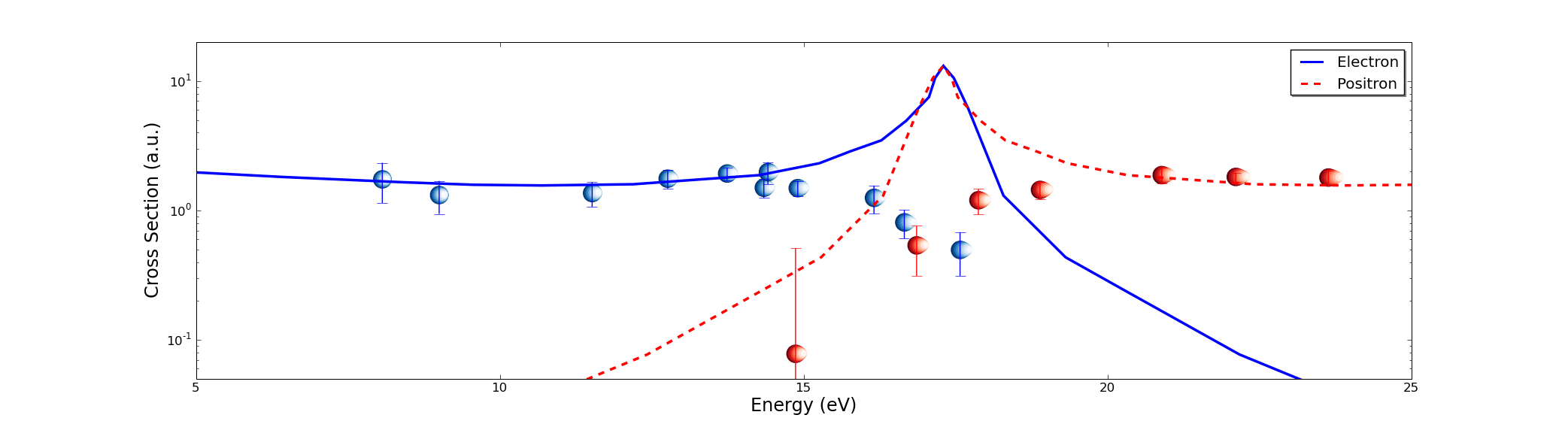# custom symbols for scatter

Hi, I am trying to put a sphere (rather than circles or disks) as markers for a
plot. I am a newbiew so I tried the poor man approach: I plotted several circles
one over the other for each point to mimic a sphere (looking it from very far).
It kind of worked, except that all are not exactly the same and they distort
when I change the aspect ratio of the plot. I am attaching two figures
to show what I mean.
I looked around in the mailing list and searched all over the web but did not
find nothing mentioned. Any help is appreciated.
Regards,
JuanCan you post a self-contained version of your code so that we can see exactly how you make the spheres? You can just use random/hardcoded numbers for the data in the plot.

Ryan

···

On Mon, Apr 27, 2009 at 7:50 AM, Juan Fiol <fiolj@…9…> wrote:

Hi, I am trying to put a sphere (rather than circles or disks) as markers for a

plot. I am a newbiew so I tried the poor man approach: I plotted several circles

one over the other for each point to mimic a sphere (looking it from very far).

It kind of worked, except that all are not exactly the same and they distort

when I change the aspect ratio of the plot. I am attaching two figures

to show what I mean.

I looked around in the mailing list and searched all over the web but did not

find nothing mentioned. Any help is appreciated.

Ryan May
School of Meteorology
University of Oklahoma

Hi, thanks Ryan for the interest. Here is a short script to produce the data. The method also breaks if I change too much the radius of the sphere or the size at which they are plotted.

I am using:
Python 2.5.4
Matplotlib 0.98.5.2

Regards,
Code follows:

···

###############################################################
#!/usr/bin/env python
from matplotlib import pyplot as plt
import matplotlib.mlab as mlab
import numpy as np
NN=9

xmin=0.1
xmax=3.5
x= np.arange(xmin,xmax,.10)
y=np.square(np.sin(x))

# Creamos la figura
fig = plt.figure()
ax.set_yscale('log')

ylimits=(0.01,1.2)

for x1,y1 in zip(x,y):

ax.set_xlim(xmin,xmax)
ax.set_ylim(ylimits)

plt.show()
###############################################################

--- On Mon, 4/27/09, Ryan May <rmay31@...287...> wrote:

From: Ryan May <rmay31@...287...>
Subject: Re: [Matplotlib-users] custom symbols for scatter
To: fiolj@...9...
Cc: matplotlib-users@lists.sourceforge.net
Date: Monday, April 27, 2009, 5:04 PM
On Mon, Apr 27, 2009 at 7:50 AM, Juan Fiol > <fiolj@...9...> wrote:

> Hi, I am trying to put a sphere (rather than circles
or disks) as markers
> for a
> plot. I am a newbiew so I tried the poor man approach:
I plotted several
> circles
> one over the other for each point to mimic a sphere
(looking it from very
> far).
> It kind of worked, except that all are not exactly the
same and they
> distort
> when I change the aspect ratio of the plot. I am
attaching two figures
> to show what I mean.
> I looked around in the mailing list and searched all
over the web but did
> not
> find nothing mentioned. Any help is appreciated.
>

Can you post a self-contained version of your code so that
we can see
exactly how you make the spheres? You can just use
random/hardcoded numbers
for the data in the plot.

Ryan

--
Ryan May